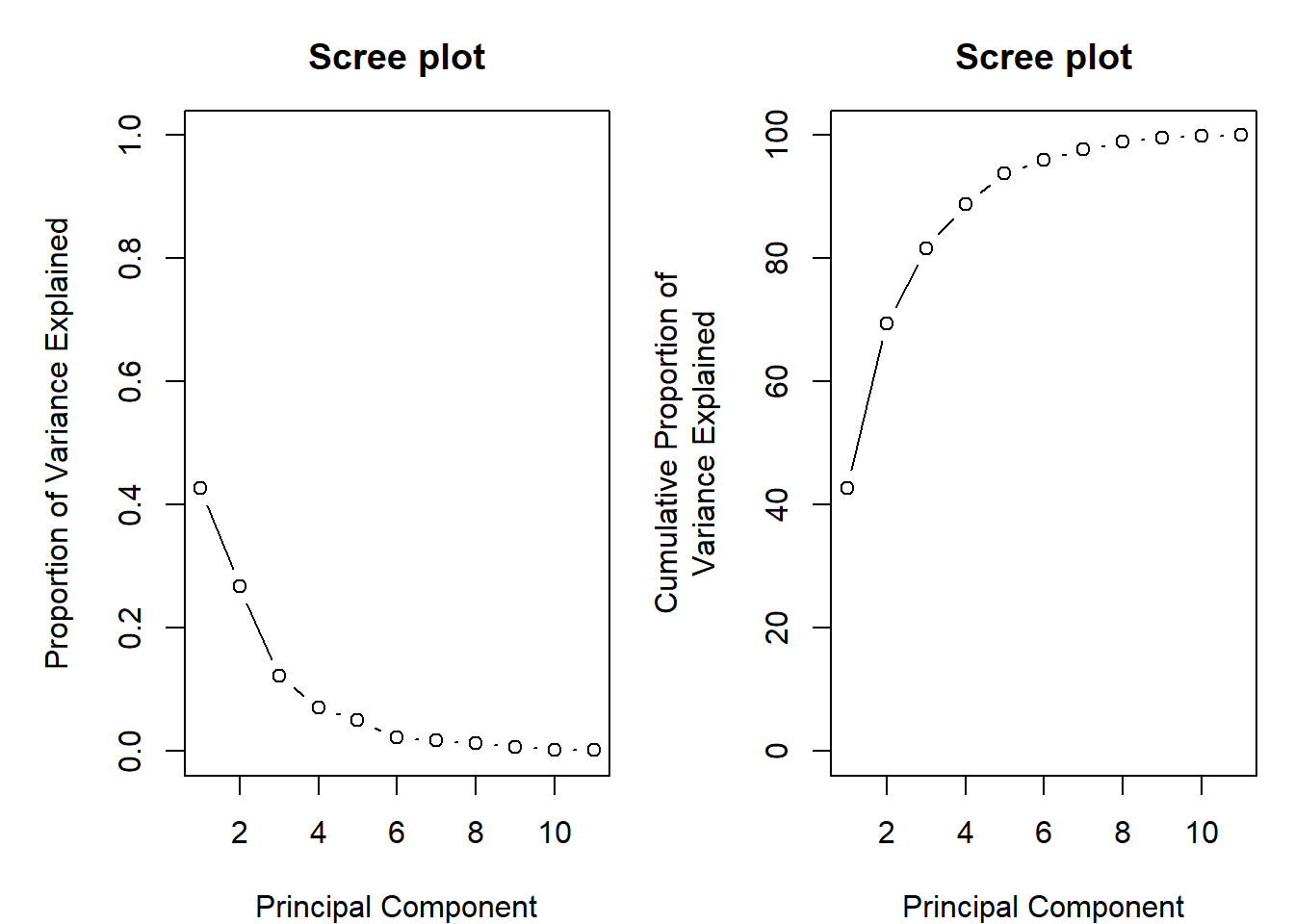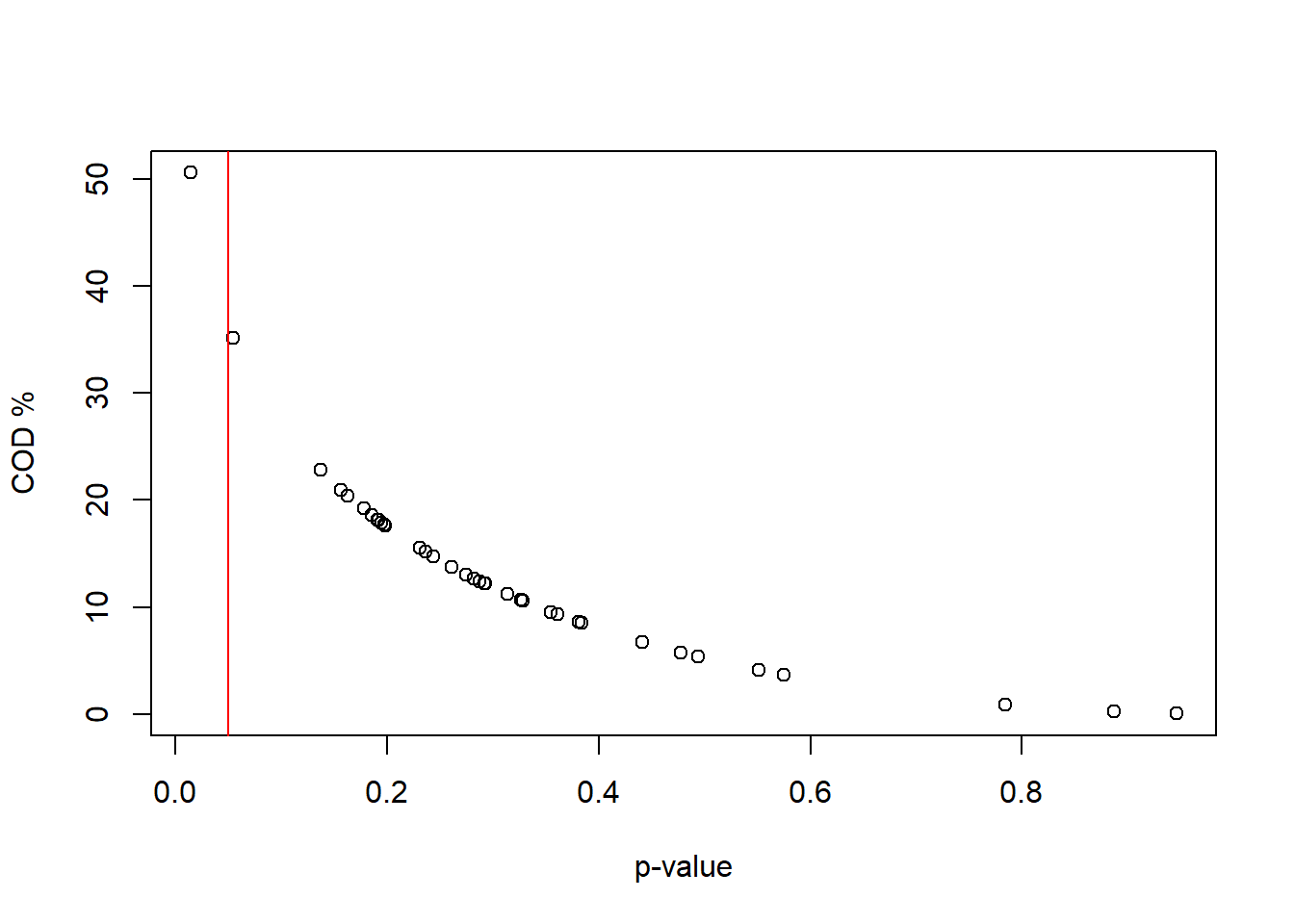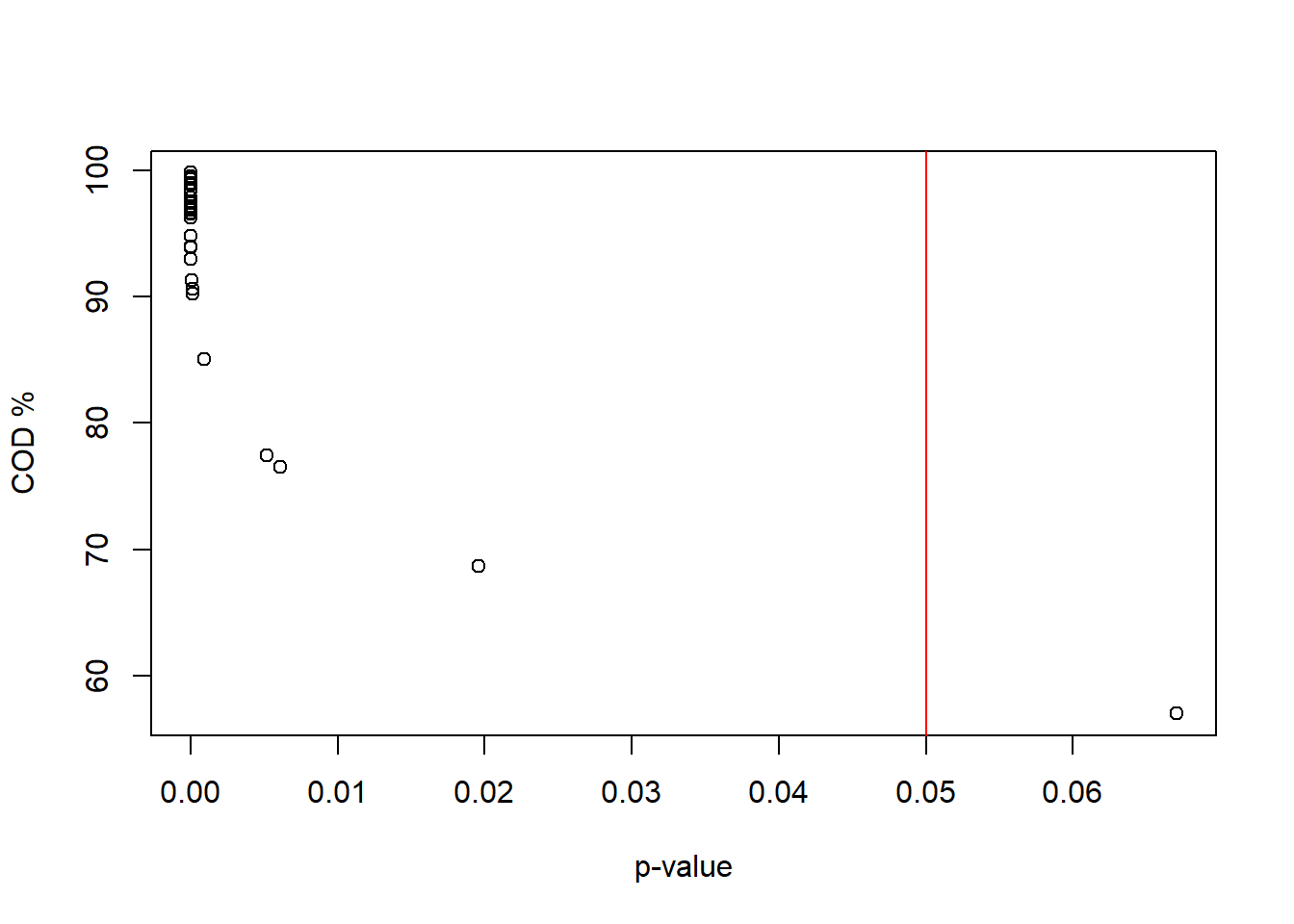We explored a climate data set from France using Multiple Linear Regression and Principal Component Analysis. Now, we want to apply a Factor Analysis on the same data set.

# data download
clim <- read.csv("https://userpage.fu-berlin.de/soga/data/raw-data/Climfrance.csv", sep = ";")

# deletion of thousands separator and formatting of non-numeric variables
clim[, "p_mean"] <- as.numeric(gsub(",", "", clim[, "p_mean"]))
clim[, "altitude"] <- as.numeric(gsub(",", "", clim[, "altitude"]))

Exercise 1: Perform a Factor analysis for the first three principal components and try to interpret them in terms of climate factors.

Principal Components
Let us look first on the principal components and evaluate the proportion of variance explained by them.

### your code here...
Show code
clim_pca <- prcomp(clim[, 2:ncol(clim)], scale. = TRUE, center = TRUE)
eig <- matrix(rep(1, 33), ncol = 11, nrow = 3)

for (i in 1:11) {
eig[1, i] <- sum(clim_pca$sdev[i]^2) } # eigenvalues for (i in 1:11) { eig[2, i] <- 100 * sum(clim_pca$sdev[i]^2) / 11
} # proportional variance

for (i in 1:11) {
eig[3, i] <- sum(eig[2, 1:i])
} # cumulative variance

rownames(eig) <- c("Eigenvalue", "Proport_Variance", "Cumulative Var")
par(mfrow = c(1, 2), mar = c(4, 5, 3, 1))
plot(eig[1, ] / 11,
xlab = "Principal Component",
ylab = "Proportion of Variance Explained",
ylim = c(0, 1),
type = "b",
main = "Scree plot"
)

plot(eig[3, ],
xlab = "Principal Component",
ylab = "Cumulative Proportion of\nVariance Explained",
ylim = c(0, 100),
type = "b",
main = "Scree plot"
)As we see in the scree plot, the first three principal components explain $$82 \%$$ of the total variance in the data. Therefore, choosing three factors for the factor analysis is reasonable.

Factor estimation

Now, let us perform the factor analysis for three factors.

### your code here...

Factor analysis:
Show code
clim_fa <- factanal(clim[, 2:ncol(clim)], factors = 3, scores = "regression", rotation = "varimax")
clim_fa
##
## Call:
## factanal(x = clim[, 2:ncol(clim)], factors = 3, scores = "regression",     rotation = "varimax")
##
## Uniquenesses:
##          altitude               lat               lon            t_mean
##             0.075             0.005             0.608             0.005
##             t_max             t_min       relhumidity            p_mean
##             0.302             0.184             0.380             0.630
##          p_max24h         rainydays sun_shine_hperyrs
##             0.459             0.005             0.234
##
##                   Factor1 Factor2 Factor3
## altitude           0.207  -0.919   0.193
## lat               -0.941          -0.331
## lon                0.435          -0.450
## t_mean             0.209   0.973
## t_max              0.124   0.819   0.108
## t_min              0.231   0.844  -0.224
## relhumidity       -0.782
## p_mean                    -0.113   0.595
## p_max24h           0.706   0.182
## rainydays         -0.863  -0.238   0.441
## sun_shine_hperyrs  0.850   0.120  -0.169
##
##                Factor1 Factor2 Factor3
## Proportion Var   0.346   0.299   0.092
## Cumulative Var   0.346   0.646   0.738
##
## Test of the hypothesis that 3 factors are sufficient.
## The chi square statistic is 84.32 on 25 degrees of freedom.
## The p-value is 2.37e-08

According to the chi-squared test, the first three PC’s are sufficient to explain the variance in the data. Clearly, the mean precipitation and maximum precipitation within 24 hours are not well explained by these factors.

Factor 1 is strongly related to sunshine hours per year and maximum precipitation within 24 hours, and highly negative related to the number of rainy days, relative humidity and latitude. This indicates that latitude is a climate determinant for those variables.

Factor 2 is strongly related with maximum, minimum and mean temperature as well as highly negative related with altitude, showing that temperature decreases with increasing height above sea level. Hence, this factor just confirms the global relation between altitude and temperature.

Factor 3 suggests a slight relation between longitude and precipitation suggesting weak effects of oceanity/continentality.

Coefficient of determination:
In order to evaluate the quality of the model, we calculate the Coefficient of Determination (COD), which is the squared correlation between values produced by the model and the observed values.

### your code here...
Show code
# model
X_new <- clim_fa$scores %*% t(clim_fa$loadings)

# coefficient of determination r²
r_var <- rep(1, 11)
for (i in 1:11) {
r_var[i] <- cor(clim[, i + 1], X_new[, i])^2
}

Qual_Var <- as.data.frame(rbind(round(clim_fa$uniquenesses, 2), round(r_var, 2))) colnames(Qual_Var) <- colnames(clim[2:ncol(clim)]) rownames(Qual_Var) <- c("Uniquenesses", "COD") Qual_Var ## altitude lat lon t_mean t_max t_min relhumidity p_mean p_max24h ## Uniquenesses 0.08 0 0.61 0 0.3 0.18 0.38 0.63 0.46 ## COD 0.93 1 0.40 1 0.7 0.82 0.62 0.38 0.54 ## rainydays sun_shine_hperyrs ## Uniquenesses 0 0.23 ## COD 1 0.77 Other than longitude and precipitation, most variables are sufficiently represented by these three factors. Let us have a look in the observation space: r_obs <- rep(1, length(clim[, 1])) for (i in seq_along(r_obs)) { r_obs[i] <- cor.test(as.numeric(clim[i, 2:ncol(clim)]), as.numeric(X_new[i, ]))$p.value
}

COD_obs <- rep(1, length(X_new[, 1]))
for (i in seq_along(COD_obs)) {
COD_obs[i] <- cor.test(as.numeric(clim[i, 2:ncol((clim))]), as.numeric(X_new[i, ]))$estimate^2 } Qual_Obs_COD <- cbind(clim$altitude, round(COD_obs, 2))
plot(r_obs, COD_obs * 100, xlab = "p-value", ylab = "COD %")
abline(v = 0.05, col = "red")Obviously, the reproduction of our observations is far beyond sufficient replicability. Let us check if we can achieve a better replicability by using a weight transformation to re-scale to the inner $$90\%-$$interval. (Weltje, 1997: eq. 13 )

Exercise 2: Can w-transformation help us to improve replicability of our observations?

Function for w-transformation

### your code here...
Show code
# function for weight transformation
w_trans <- function(x, q = 0) {
w <- matrix(NA, nrow = nrow(x), ncol = ncol(x))

for (i in 1:nrow(x)) {
for (j in 1:ncol(x)) {{ h <- quantile(x[, j], q)
g <- quantile(x[, j], 1 - q)
w[i, j] <- (x[i, j] - h) / (g - h) }}
}
colnames(w) <- colnames(x)
return(w)
}

Application of w-transformation

### your code here...
Show code
#  apply w_trans, cut off the upper and lower 5%
clim_w <- w_trans(clim[, 2:ncol(clim)], 0.05) # matrix with weight transformed data
colnames(clim_w) <- colnames(clim[, 2:ncol(clim)])

clim_fa_w <- factanal(x = clim_w, factors = 3, rotation = "varimax", scores = "regression", scale = TRUE)
clim_fa_w
##
## Call:
## factanal(x = clim_w, factors = 3, scores = "regression", rotation = "varimax",     scale = TRUE)
##
## Uniquenesses:
##          altitude               lat               lon            t_mean
##             0.075             0.005             0.608             0.005
##             t_max             t_min       relhumidity            p_mean
##             0.302             0.184             0.380             0.630
##          p_max24h         rainydays sun_shine_hperyrs
##             0.459             0.005             0.234
##
##                   Factor1 Factor2 Factor3
## altitude           0.207  -0.919   0.193
## lat               -0.941          -0.331
## lon                0.435          -0.450
## t_mean             0.209   0.973
## t_max              0.124   0.819   0.108
## t_min              0.231   0.844  -0.224
## relhumidity       -0.782
## p_mean                    -0.113   0.595
## p_max24h           0.706   0.182
## rainydays         -0.863  -0.238   0.441
## sun_shine_hperyrs  0.850   0.120  -0.169
##
##                Factor1 Factor2 Factor3
## Proportion Var   0.346   0.299   0.092
## Cumulative Var   0.346   0.646   0.738
##
## Test of the hypothesis that 3 factors are sufficient.
## The chi square statistic is 84.32 on 25 degrees of freedom.
## The p-value is 2.37e-08

Back-transformation and evaluation:
Show code
X_new_w <- clim_fa_w$scores %*% t(clim_fa_w$loadings) # model

# COD of the variables between the data and the new model

r_var <- rep(1, 11)
for (i in 1:11) {
r_var[i] <- cor(clim_w[, i], X_new_w[, i])^2
}

Qual_Var <- as.data.frame(rbind(round(clim_fa_w$uniquenesses, 2), round(r_var, 2))) colnames(Qual_Var) <- colnames(clim[2:ncol(clim)]) rownames(Qual_Var) <- c("Uniquenesses", "COD") Qual_Var ## altitude lat lon t_mean t_max t_min relhumidity p_mean p_max24h ## Uniquenesses 0.08 0 0.61 0 0.3 0.18 0.38 0.63 0.46 ## COD 0.93 1 0.40 1 0.7 0.82 0.62 0.38 0.54 ## rainydays sun_shine_hperyrs ## Uniquenesses 0 0.23 ## COD 1 0.77 Because w-transformation just translates and scales, there are no surprises concerning the variable reproduction. We still have an extraordinary high uniqueness in longitude and precipitation. # back-transformation X_new_b <- matrix(NA, nrow = nrow(clim_w), ncol = ncol(clim_w)) q <- 0.05 for (i in 1:nrow(clim_w)) { for (j in 1:ncol(clim_w)) { h <- quantile(clim[, j + 1], q) g <- quantile(clim[, j + 1], 1 - q) X_new_b[i, j] <- X_new_w[i, j] * (g - h) + h } } colnames(X_new_b) <- colnames(clim_w) ###### reproducibility of observations after back-transformation ##### r_obs_b <- rep(1, length(X_new_b[, 1])) for (i in seq_along(r_obs_b)) { r_obs_b[i] <- cor.test(as.numeric(clim[i, 2:ncol((clim))]), as.numeric(X_new_b[i, ]))$p.value
}

Qual_Obs <- cbind(clim$altitude, round(r_obs_b, 2)) COD_obs_b <- rep(1, length(X_new_b[, 1])) for (i in seq_along(COD_obs_b)) { COD_obs_b[i] <- cor.test(as.numeric(clim[i, 2:ncol((clim))]), as.numeric(X_new_b[i, ]))$estimate
}

Qual_Obs_COD <- cbind(clim\$altitude, round(COD_obs_b, 2))
plot(r_obs_b, COD_obs_b * 100, xlab = "p-value", ylab = "COD %")
abline(v = 0.05, col = "red")After applying w-transformation and transforming back we find that our model becomes remarkably better in reproducing the original data.

Citation

The E-Learning project SOGA-R was developed at the Department of Earth Sciences by Kai Hartmann, Joachim Krois and Annette Rudolph. You can reach us via mail by soga[at]zedat.fu-berlin.de.You may use this project freely under the Creative Commons Attribution-ShareAlike 4.0 International License.

Please cite as follow: Hartmann, K., Krois, J., Rudolph, A. (2023): Statistics and Geodata Analysis using R (SOGA-R). Department of Earth Sciences, Freie Universitaet Berlin.